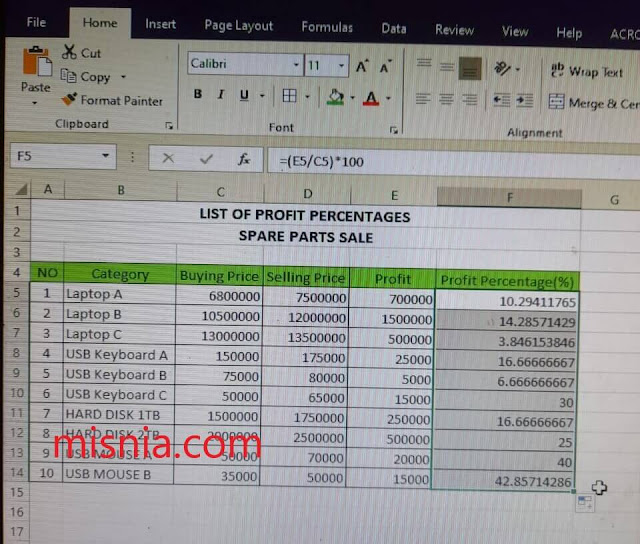# Percentage Formula in Excel Automatically

The percentage formula in excel ms office we can use to calculate percentage numbers automatically without the need to calculate them manually

We can use this formula to calculate any percentage, both the percentage of division, discount, and other percentage formula calculations.

Whatever your needs are related to percentages, we can use this percentage formula in excel, even this method can automatically provide the results for us. So, in the tutorial below, misnia will share with you about this magic formula for the readers.

## Percentage Formula in Excel Automatically?

To calculate percentages in Excel, we can actually do it manually using the percent formula, which of course we learned this formula when we were in school, namely Percentage (%) = (number of parts)/(total amount) X 100.

That's not true, but the formula above is a manual formula, friends.

Especially for those of you who want to calculate percentages automatically in Microsoft Excel, you can try using the following formula below.

## Percentage Formula in Excel Automatically

In practice, to calculate percentages in Excel automatically, we still use the manual percentage calculation formula as above, but we will look for automatic steps to make our work easier in calculating percentages.

Alright, let's just go without a length, below is a complete step-by-step tutorial as follows.

• Prepare data in the form of values in one cell that we will take the percentage
• And also prepare a special column for the percentage value that will appear automatically
• In the column for percentage, type the formula exactly like the formula below

= (Cell you want to count)/(Number of percent)*100

See below picture for detail

• After you write the formula, then press Enter on the keyboard

Now, please look at the results in the percentage column, we automatically get the numbers from the percentages we expect. We can tinker with the arrangement of this formula as needed and based on the data we want to find.

If this formula is in accordance with the needs you mean?

Well, my dear misnia readers, we still have another formula that is still related to percentages, which is to calculate the percentage of other data without the need to recreate it. Read more in the excel tutorial below

• Click on the percentage result cell that you created earlier
• Move the cursor to the lower right corner of the cell until the cursor changes to a black + symbol• If it is according to the instructions above, then double click or drag down until the last line of data we want to find the percentageAfter the above steps are complete, now we have the percentage data for all values, very easy right?